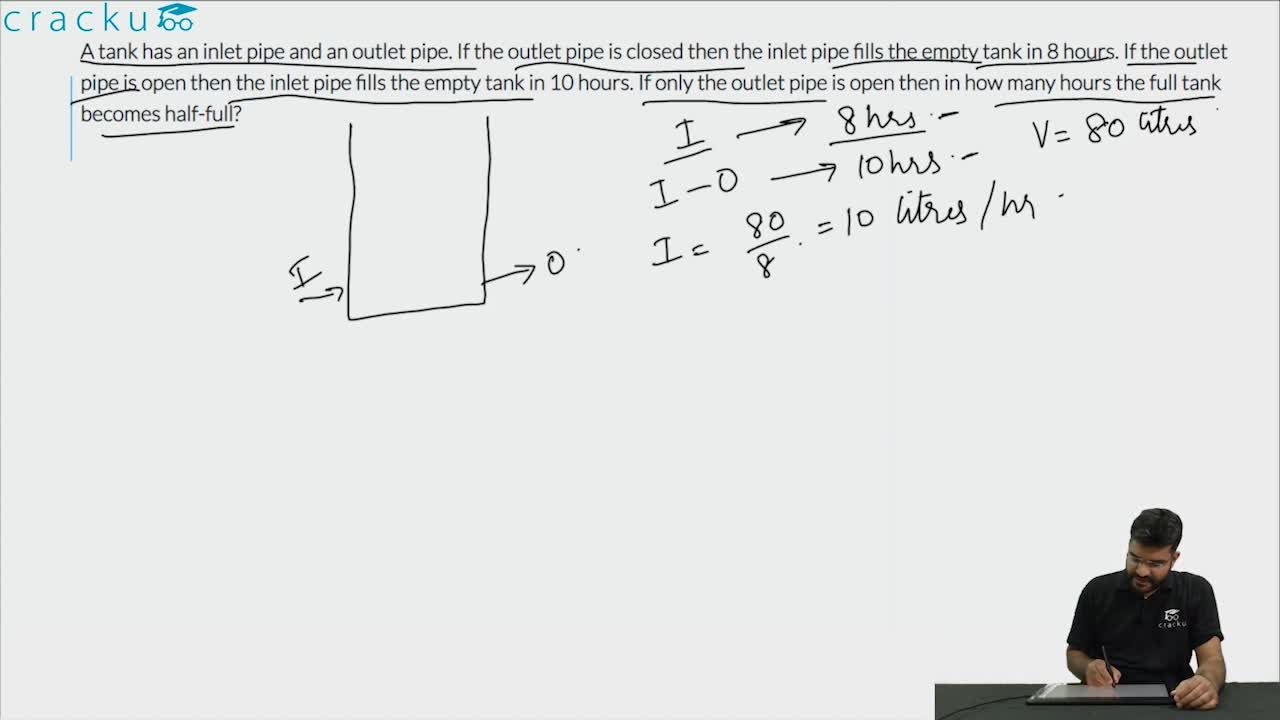Question 74

# A tank has an inlet pipe and an outlet pipe. If the outlet pipe is closed then the inlet pipe fills the empty tank in 8 hours. If the outlet pipe is open then the inlet pipe fills the empty tank in 10 hours. If only the outlet pipe is open then in how many hours the full tank becomes half-full?

Solution

Let the time taken by the outlet pipe to empty = x hours
Then, $$\frac{1}{8} - \frac{1}{x} = \frac{1}{10}$$
=> $$x = 40$$
Hence time taken by the outlet pipe to make the tank half-full = 40/2 = 20 hour

### View Video Solution